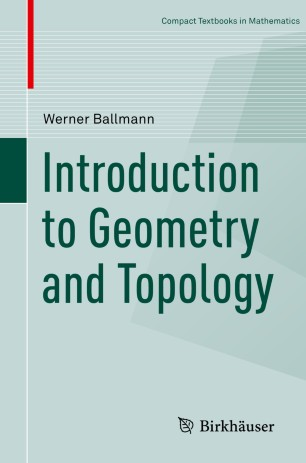# Introduction to Geometry and Topology

• Werner BallmannTextbook

Part of the Compact Textbooks in Mathematics book series (CTM)

1. Front Matter
Pages i-x
2. Werner Ballmann
Pages 1-25
3. Werner Ballmann
Pages 27-67
4. Werner Ballmann
Pages 69-101
5. Werner Ballmann
Pages 103-152
6. Back Matter
Pages 153-169

### Introduction

This book provides an introduction to topology, differential topology, and differential geometry. It is based on manuscripts refined through use in a variety of lecture courses. The first chapter covers elementary results and concepts from point-set topology. An exception is the Jordan Curve Theorem, which is proved for polygonal paths and is intended to give students a first glimpse into the nature of deeper topological problems.

The second chapter of the book introduces manifolds and Lie groups, and examines a wide assortment of examples. Further discussion explores tangent bundles, vector bundles, differentials, vector fields, and Lie brackets of vector fields. This discussion is deepened and expanded in the third chapter, which introduces the de Rham cohomology and the oriented integral and gives proofs of the Brouwer Fixed-Point Theorem, the Jordan-Brouwer Separation Theorem, and Stokes's integral formula.

The fourth and final chapter is devoted to the fundamentals of differential geometry and traces the development of ideas from curves to submanifolds of Euclidean spaces. Along the way, the book discusses connections and curvature--the central concepts of differential geometry. The discussion culminates with the Gauß equations and the version of Gauß's theorema egregium for submanifolds of arbitrary dimension and codimension.

This book is primarily aimed at advanced undergraduates in mathematics and physics and is intended as the template for a one- or two-semester bachelor's course.

### Keywords

field cohomology curvature curv Liesche Group plurality context

#### Authors and affiliations

• Werner Ballmann
• 1
1. 1.Max-Planck-Institut für MathematikBonnGermany